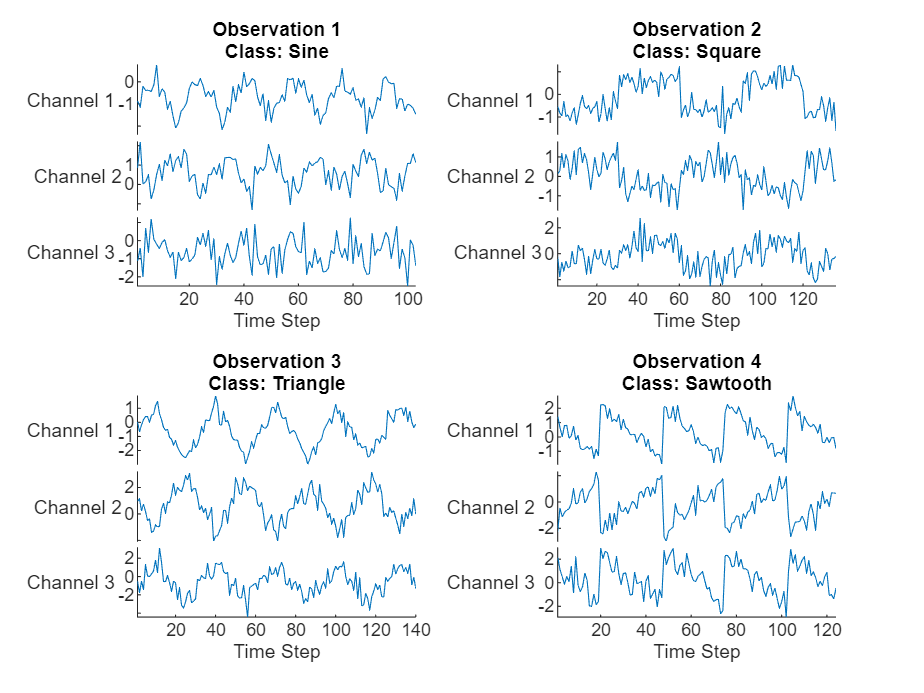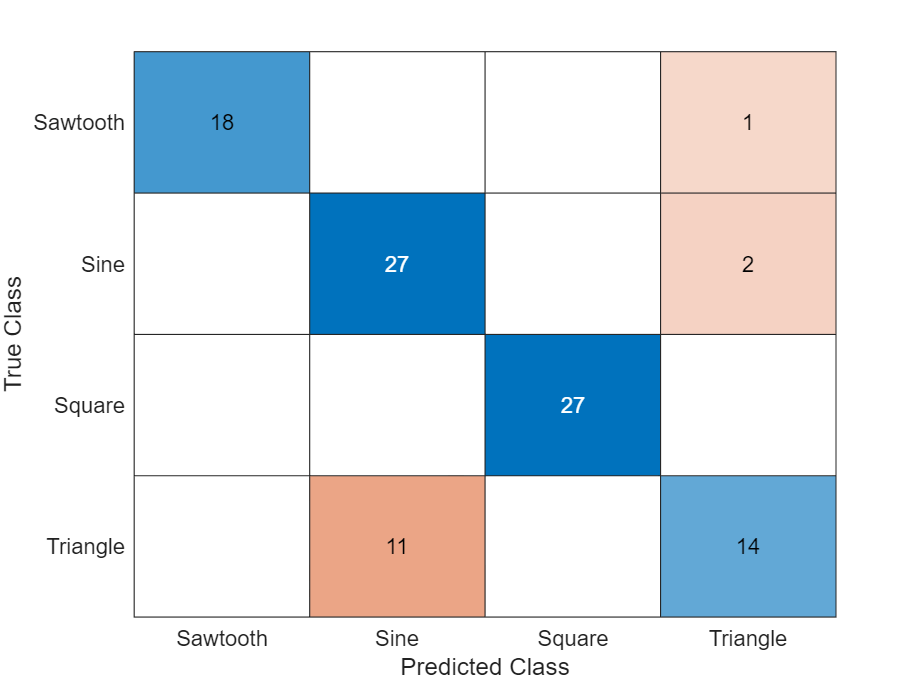# Train Sequence Classification Network Using Custom Training Loop

This example shows how to train a network that classifies sequences with a custom learning rate schedule.

You can train most types of neural networks using the `trainNetwork` and `trainingOptions` functions. If the `trainingOptions` function does not provide the options you need (for example, a custom learning rate schedule), then you can define your own custom training loop using `dlarray` and `dlnetwork` objects for automatic differentiation. For an example showing how to train a convolutional neural network for sequence classification using the `trainNetwork` function, see Sequence Classification Using 1-D Convolutions.

Training a network in a custom training loop with sequence data requires some additional processing steps when compared with image or feature data. Most deep learning functions require data passed as numeric arrays with a fixed sequence length. If you have sequence data where observations have varying lengths, then you must pad or truncate the sequences in each mini-batch so that they have the same length.

This example trains a network to classify sequences with the time-based decay learning rate schedule: for each iteration, the solver uses the learning rate given by ${\rho }_{\mathit{t}}=\frac{{\rho }_{0}}{1+\mathit{k}\text{\hspace{0.17em}}\mathit{t}}$, where t is the iteration number, ${\rho }_{0}$ is the initial learning rate, and k is the decay.

Load the Waveform data set from `WaveformData.mat`. The observations are `numChannels`-by-`numTimeSteps` arrays, where `numChannels` and `numTimeSteps` are the number of channels and time steps of the sequence, respectively. The sequences have different lengths.

`load WaveformData`

View the sizes of the first few sequences.

`data(1:5)`
```ans=5×1 cell array {3×103 double} {3×136 double} {3×140 double} {3×124 double} {3×127 double} ```

View the number of channels. To train the network, each sequence must have the same number of channels.

`numChannels = size(data{1},1)`
```numChannels = 3 ```

Visualize the first few sequences in a plot.

```figure tiledlayout(2,2) for i = 1:4 nexttile stackedplot(data{i}',DisplayLabels="Channel " + (1:numChannels)); title("Observation " + i + newline + "Class: " + string(labels(i))) xlabel("Time Step") end```Determine the number of classes in the training data.

```classes = categories(labels); numClasses = numel(classes);```

Partition the data into training and test partitions. Train the network using the 90% of the data and set aside 10% for testing.

```numObservations = numel(data); idxTrain = 1:floor(0.9*numObservations); XTrain = data(idxTrain); TTrain = labels(idxTrain); idxTest = floor(0.9*numObservations)+1:numObservations; XTest = data(idxTest); TTest = labels(idxTest);```

### Define Network

Define the network for sequence classification.

• For the sequence input, specify a sequence input layer with input size matching the number of channels of the training data.

• Specify three convolution-layernorm-ReLU blocks.

• Pad the input to the convolution layers such that the output has the same size by setting the `Padding` option to `"same"`.

• For the first convolution layer specify 20 filters of size 5.

• Pool the timesteps to a single value using a 1-D global average pooling layer.

• For classification, specify a fully connected layer with size matching the number of classes

• To map the output to probabilities, include a softmax layer.

When training a network using a custom training loop, do not include an output layer.

```layers = [ sequenceInputLayer(numChannels) convolution1dLayer(5,20,Padding="same") layerNormalizationLayer reluLayer convolution1dLayer(5,20,Padding="same") layerNormalizationLayer reluLayer convolution1dLayer(5,20,Padding="same") layerNormalizationLayer reluLayer globalAveragePooling1dLayer fullyConnectedLayer(numClasses) softmaxLayer];```

Create a `dlnetwork` object from the layer array.

`net = dlnetwork(layers)`
```net = dlnetwork with properties: Layers: [13×1 nnet.cnn.layer.Layer] Connections: [12×2 table] Learnables: [14×3 table] State: [0×3 table] InputNames: {'sequenceinput'} OutputNames: {'softmax'} Initialized: 1 View summary with summary. ```

### Define Model Loss Function

Training a deep neural network is an optimization task. By considering a neural network as a function $f\left(X;\theta \right)$, where $X$ is the network input, and $\theta$ is the set of learnable parameters, you can optimize $\theta$ so that it minimizes some loss value based on the training data. For example, optimize the learnable parameters $\theta$ such that for a given inputs $X$ with a corresponding targets $T$, they minimize the error between the predictions $Y=f\left(X;\theta \right)$ and $T$.

Create the function `modelLoss`, listed in the Model Loss Function section of the example, that takes as input the `dlnetwork` object, a mini-batch of input data with corresponding targets, and returns the loss, the gradients of the loss with respect to the learnable parameters, and the network state.

### Specify Training Options

Train for 60 epochs with a mini-batch size of 128.

```numEpochs = 60; miniBatchSize = 128;```

Specify the options for Adam optimization. Specify an initial learn rate of 0.005 with a decay of 0.01.

```initialLearnRate = 0.005; learnRateDecay = 0.01;```

### Train Model

Create a `minibatchqueue` object that processes and manages mini-batches of data during training.

Mini-batch queue objects require data specified as datastores. Convert the sequences and labels to array datastores and combine them using the `combine` function. To output sequences as a cell array of numeric arrays, specify an output type of `"same"` for the sequence data.

```adsXTrain = arrayDatastore(XTrain,OutputType="same"); adsTTrain = arrayDatastore(TTrain); cdsTrain = combine(adsXTrain,adsTTrain);```

Create a `minibatchqueue` object that processes and manages mini-batches of data during training. For each mini-batch:

• Use the custom mini-batch preprocessing function `preprocessMiniBatch` (defined at the end of this example) to pad the sequences to have the same length and convert the labels to one-hot encoded variables.

• Format the sequence data with the dimension labels `"CTB"` (channel, time, batch). By default, the `minibatchqueue` object converts the data to `dlarray` objects with underlying type `single`. Do not format the class labels.

• Train on a GPU if one is available. By default, the `minibatchqueue` object converts each output to a `gpuArray` if a GPU is available. Using a GPU requires Parallel Computing Toolbox™ and a supported GPU device. For information on supported devices, see GPU Computing Requirements (Parallel Computing Toolbox).

```mbq = minibatchqueue(cdsTrain,... MiniBatchSize=miniBatchSize,... MiniBatchFcn=@preprocessMiniBatch,... MiniBatchFormat=["CTB" ""]);```

```averageGrad = []; averageSqGrad = [];```

Calculate the total number of iterations for the training progress monitor.

```numObservationsTrain = size(XTrain,1); numIterationsPerEpoch = ceil(numObservationsTrain / miniBatchSize); numIterations = numEpochs * numIterationsPerEpoch;```

Initialize the `TrainingProgressMonitor` object. Because the timer starts when you create the monitor object, make sure that you create the object close to the training loop.

```monitor = trainingProgressMonitor( ... Metrics="Loss", ... Info=["Epoch","LearnRate"], ... XLabel="Iteration");```

Train the network using a custom training loop. For each epoch, shuffle the data and loop over mini-batches of data. For each mini-batch:

• Evaluate the model loss and gradients using the `dlfeval` and `modelLoss` functions.

• Determine the learning rate for the time-based decay learning rate schedule.

• Update the network parameters using the `adamupdate` function.

• Update the loss, learn rate, and epoch values in the training progress monitor.

• Stop if the Stop property is true. The `Stop` property value of the `TrainingProgressMonitor` object changes to true when you click the Stop button.

```epoch = 0; iteration = 0; % Loop over epochs. while epoch < numEpochs && ~monitor.Stop epoch = epoch + 1; % Shuffle data. shuffle(mbq); % Loop over mini-batches. while hasdata(mbq) && ~monitor.Stop iteration = iteration + 1; % Read mini-batch of data. [X,T] = next(mbq); % Evaluate the model gradients and loss using dlfeval and the % modelLoss function. [loss,gradients] = dlfeval(@modelLoss,net,X,T); % Determine learning rate for time-based decay learning rate schedule. learnRate = initialLearnRate/(1 + learnRateDecay*iteration); % Update the network parameters using the Adam optimizer. [net,averageGrad,averageSqGrad] = adamupdate(net,gradients, ... averageGrad,averageSqGrad,iteration,learnRate); % Update the training progress monitor. recordMetrics(monitor,iteration,Loss=loss); updateInfo(monitor,Epoch=epoch,LearnRate=learnRate); monitor.Progress = 100 * iteration/numIterations; end end```### Test Model

Test the classification accuracy of the model by comparing the predictions on the test set with the targets.

After training, making predictions on new data does not require the labels. Create `minibatchqueue` object containing only the predictors of the test data:

• Specify the same mini-batch size used for training.

• Preprocess the predictors using the `preprocessMiniBatchPredictors` function, listed at the end of the example.

• For the single output of the datastore, specify the mini-batch format `"CTB"` (channel, time, batch).

```adsXTest = arrayDatastore(XTest,OutputType="same"); mbqTest = minibatchqueue(adsXTest, ... MiniBatchSize=miniBatchSize, ... MiniBatchFcn=@preprocessMiniBatchPredictors, ... MiniBatchFormat="CTB");```

Loop over the mini-batches and classify the sequences using `modelPredictions` function, listed at the end of the example.

`YTest = modelPredictions(net,mbqTest,classes);`

Evaluate the classification accuracy.

`accuracy = mean(TTest == YTest)`
```accuracy = 0.8200 ```

Visualize the predictions in a confusion chart.

```figure confusionchart(TTest,YTest)```Large values on the diagonal indicate accurate predictions for the corresponding class. Large values on the off-diagonal indicate strong confusion between the corresponding classes.

### Supporting Functions

#### Model Loss Function

The `modelLoss` function takes a `dlnetwork` object `net`, a mini-batch of input data `X` with corresponding targets `T` and returns the loss and the gradients of the loss with respect to the learnable parameters in `net`. To compute the gradients automatically, use the `dlgradient` function.

```function [loss,gradients] = modelLoss(net,X,T) % Forward data through network. Y = forward(net,X); % Calculate cross-entropy loss. loss = crossentropy(Y,T); % Calculate gradients of loss with respect to learnable parameters. gradients = dlgradient(loss,net.Learnables); end```

#### Model Predictions Function

The `modelPredictions` function takes a `dlnetwork` object `net`, a `minibatchqueue` of input data `mbq`, and the network classes, and computes the model predictions by iterating over all data in the `minibatchqueue` object. The function uses the `onehotdecode` function to find the predicted class with the highest score.

```function Y = modelPredictions(net,mbq,classes) Y = []; % Loop over mini-batches. while hasdata(mbq) X = next(mbq); % Make prediction. scores = predict(net,X); % Decode labels and append to output. labels = onehotdecode(scores,classes,1)'; Y = [Y; labels]; end end```

#### Mini Batch Preprocessing Function

The `preprocessMiniBatch` function preprocesses a mini-batch of predictors and labels using the following steps:

1. Preprocess the sequences using the `preprocessMiniBatchPredictors` function.

2. Extract the label data from the incoming cell array and concatenate into a categorical array along the second dimension.

3. One-hot encode the categorical labels into numeric arrays. Encoding into the first dimension produces an encoded array that matches the shape of the network output.

```function [X,T] = preprocessMiniBatch(dataX,dataT) % Preprocess predictors. X = preprocessMiniBatchPredictors(dataX); % Extract label data from cell and concatenate. T = cat(2,dataT{1:end}); % One-hot encode labels. T = onehotencode(T,1); end```

#### Mini-Batch Predictors Preprocessing Function

The `preprocessMiniBatchPredictors` function preprocesses a mini-batch of predictors by padding the sequence data in the input cell array over the second (time) dimension. The function returns the data as a `numChannels`-by-`numTimeSteps`-by-`numObservations` array. To pass this information to downstream functions, specify that this data has a format of `"CTB"` (channel, time, batch).

```function X = preprocessMiniBatchPredictors(dataX) % Pad sequences. X = padsequences(dataX,2); end```